Next: Hierarchical models Up: Bayesian statistics Previous: Bayesian statistics   Contents

## Constructing probabilistic models

To use the techniques of probabilistic modelling, one must usually somehow specify the likelihood of the data given some parameters. Not all models are, however, probabilistic in nature and have naturally emerging likelihoods. Many models are defined as generative models by stating how the observed data could be generated from unknown parameters or latent variables.

For given data vectors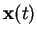, a simple linear generative model could be written as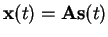(3.4)

where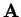is an unobserved transformation matrix and vectors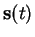are unobserved hidden or latent variables. In some application areas the latent variables are also called sources or factors [6,35].

One way to turn such a generative model into a probabilistic model is to add a noise term to the Equation (3.4). This yields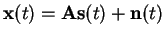(3.5)

where the noise can, for example, be assumed to be zero-mean and Gaussian as in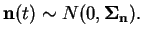(3.6)

Here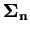denotes the covariance matrix of the noise and it is usually assumed to be diagonal to make the different components of the noise independent.

This implies a likelihood for the data given by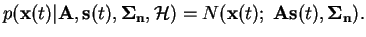(3.7)

For a complete probabilistic model one also needs priors for all the parameters of the model. For instance hierarchical models and conjugate priors can be useful mathematical tools here but in the end the priors should be chosen to represent true prior knowledge on the solution of the problem at hand.Next: Hierarchical models Up: Bayesian statistics Previous: Bayesian statistics   Contents
Antti Honkela 2001-05-30